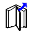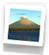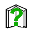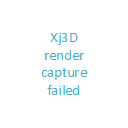## X3D Example Archives: X3D4WA, X3D for Web Authors, Chapter 13 Geometry Triangles Quadrilaterals

 Triangles and quadrilaterals are fundamental geometry representations that are typically created by authoring tools to build complex polygonal meshes and shapes. Low-level high-performance nodes for geometry definition and fast rendering include TriangleSet, TriangleFanSet, TriangleStripSet, IndexedTriangleSet, IndexedTriangleFanSet, IndexedTriangleStripSet, QuadSet and IndexedQuadSet. Special definition rules apply to each node in order to best match underlying graphics hardware requirements. Each is placed individually inside a Shape node. The supporting Chapter 13 slideset and course videos (NPS, YouTube) for X3D for Web Authors are available online via X3dGraphics.com. Also available: X3D Scene Authoring Hints: Meshes.12 X3D Models       X3D Model DescriptionsIndexed Quad Set Example A simple example of the use of the IndexedQuadSet node.Indexed Triangle Fan Set Example A simple example of the use of the IndexedTriangleFanSet node.Indexed Triangle Set Example A simple example of the use of the IndexedTriangleSet node.Indexed Triangle Strip Set Example An example of the use of the IndexedTriangleStripSet node.Morphing Triangle Set Animated example of a morphing TriangleSetNonplanar Polygons Illustration of nonplanar polygons.Normal Example A simple illustration of the Normal node on the coloring of triangles.Quad Set Example A simple example of the use of the QuadSet node.Summary Indexed Triangle Sets A collection of example usage for all IndexedTriangle*Set nodes.Triangle Fan Set Example A simple example of the use of the TriangleFanSet node.Triangle Set Example A simple example of the use of the TriangleSet node.Triangle Strip Set Example A simple example of the use of the TriangleStripSet node.